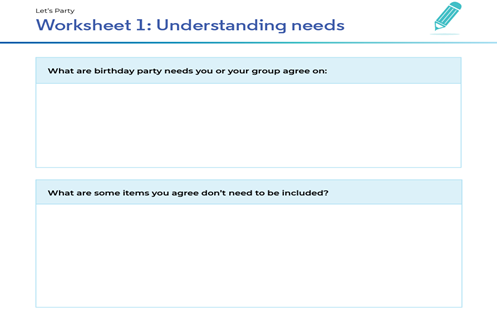# Let's party!

Students understand and compare data, they grasp the notion of budgeting and appreciate how needs and wants vary between people and cultures.

Year level(s) Year 4
Audience Teacher
Purpose Teaching resource, Teaching strategies
Teaching strategies and pedagogical approaches Culturally responsive pedagogies
Keywords budgeting, diversity, inclusive, financial literacy

## Curriculum alignment

Strand and focus Number
Topics Mathematical modelling, Money and financial mathematics
AC: Mathematics (V9.0) content descriptions
AC9M4N08
Use mathematical modelling to solve practical problems involving additive and multiplicative situations including financial contexts; formulate the problems using number sentences and choose efficient calculation strategies, using digital tools where appropriate; interpret and communicate solutions in terms of the situation

AC9M4N01
Recognise and extend the application of place value to tenths and hundredths and use the conventions of decimal notation to name and represent decimals

AC9M4N06
Develop efficient strategies and use appropriate digital tools for solving problems involving addition and subtraction, and multiplication and division where there is no remainder

Multiplicative strategies (P6)
Understanding money (P7)